# What is longitudinal expansion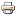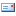### The stretching of bodies

The elongation is an indication of the relative change in length of a body under load (shortening or lengthening), for example due to a force acting on the body or due to a change in temperature (thermal expansion) *. The formula drawing is ε. If the dimensions of the body increase, this is called positive stretching or stretching, in the reverse case it is referred to as negative stretching or compression.### definition

Strain is defined as follows: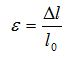l0 is the original length and ∆l is the change in length. Elongation is given as a dimensionless number or as a percentage, for this it must be multiplied by 100.

#####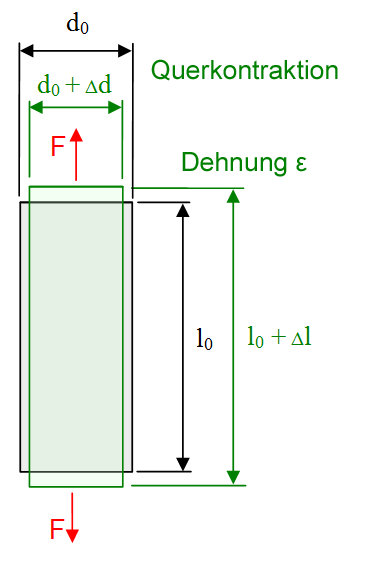Deformation - elongation & transverse contraction under tensile force

The elongation is - within certain limits - in many materials * proportional to the acting force, Hooke's law expresses this in the linear-elastic range. As a result of the transverse contraction, there is also an expansion across the direction of force, the ratio of longitudinal and transverse expansion is known as the Poisson's number.

Shear, compression and tensile forces can also occur in combination in a general scenario. The result is complex expansions in the various spatial directions, which can be fully mathematically described by tensors of the force or expansion. Computer models for deformation simulations are based on these tensors, such as the finite element method.

### The technical stretch

If two or more forces act on one another, two different reference systems are generally used to calculate the strain. A technical stretch is present when it reaches the initial length l0 which applies before the first force is applied. This will make l0 a constant, which makes this method particularly easy. Another name for technical stretching is Cauchy stretching
εC..

However, technical expansion has the disadvantage that it cannot be scaled, because the total sum of both partial expansions does not match the total expansion: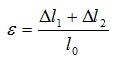is not the same as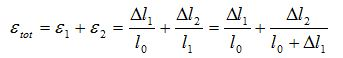.

However, as long as ∆l1 << l0 applies approximately:
l1 ≈ l0 or ε2 ≈ ∆l2/ l0 and thus ε ≈ ε1 + ε2 .

### The logarithmic expansion

Unlike technical elongation, logarithmic, true or Hencky elongation is related to the current body length, which is pre-deformed by previous forces
has been. It is defined as follows: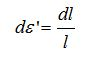and thusFrom a mathematical point of view, the technical expansion represents a series expansion of the formula for the logarithmic expansion in a Taylor series *, which breaks off after the first term. In the small expansion range there is therefore this connection between the two definitions:

ε ’≈ ε